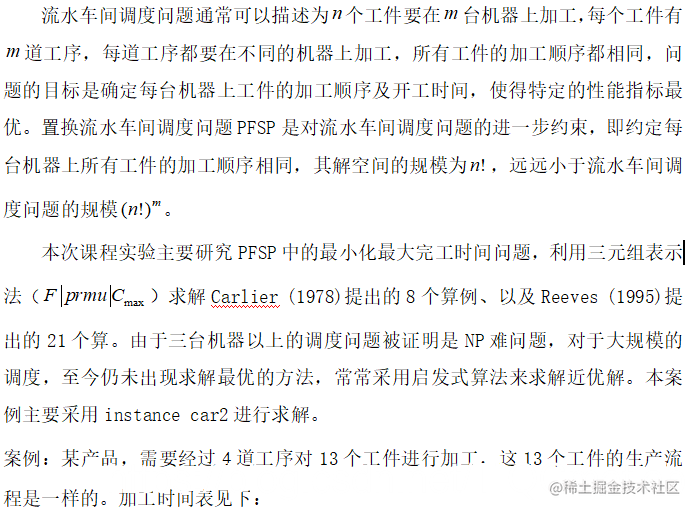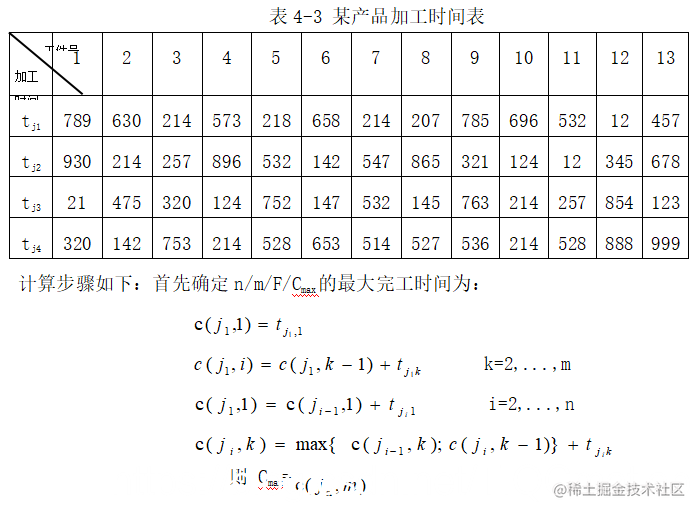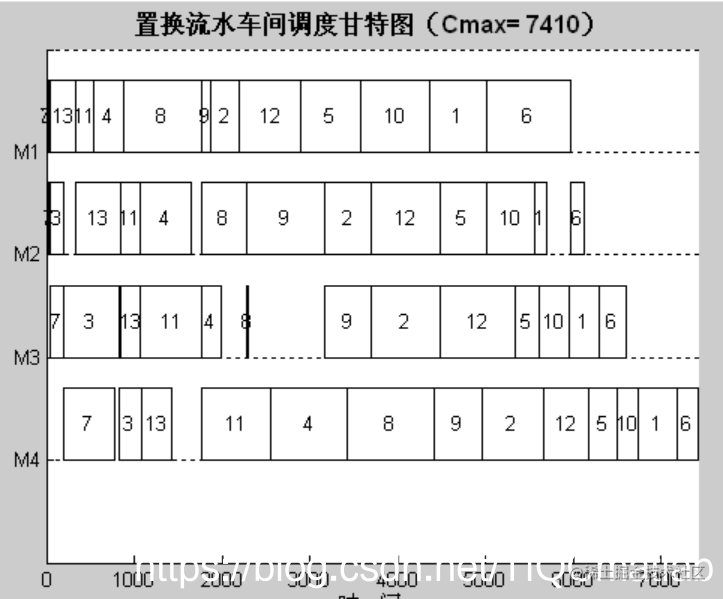# 【车间调度】基于matlab遗传算法求解置换流水车间调度问题【含Matalb源码 176期】

## 一、背景(一)

1、工件约束

2、机器约束

(l)在前面机器加工时间较短、后面机器加工时间较长的工件，安排在序列前。这样可以使得后面的机器尽快参加工作，并且后面的机器不需要作空等待，
(2)机器加工时间较为平均且加工时间较长的工件，安排在序列的中部。这样可以使得各个机器在中期的时候都能得到运作。
(3〕前面加工时间较长，后面加一〔时间较短的上件女排在序列尾部。这样使得前面的机器能“延迟”完工，后面的机器尽快完工。

## 二、源代码

``````function [Zp,Y1p,Y2p,Y3p,Xp,LC1,LC2]=JSPGA(M,N,Pm,T,P)
%  输入参数列表
%  M       遗传进化迭代次数
%  N       种群规模(取偶数)
%  Pm      变异概率
%  T       m×n的矩阵，存储m个工件n个工序的加工时间
%  P       1×n的向量，n个工序中，每一个工序所具有的机床数目
%  输出参数列表
%  Zp      最优的Makespan值
%  Y1p     最优方案中，各工件各工序的开始时刻，可根据它绘出甘特图
%  Y2p     最优方案中，各工件各工序的结束时刻，可根据它绘出甘特图
%  Y3p     最优方案中，各工件各工序使用的机器编号
%  Xp      最优决策变量的值，决策变量是一个实数编码的m×n矩阵
%  LC1     收敛曲线1，各代最优个体适应值的记录
%  LC2     收敛曲线2，各代群体平均适应值的记录
%  最后，程序还将绘出三副图片：两条收敛曲线图和甘特图（各工件的调度时序图）
%第一步：变量初始化
[m,n]=size(T);%m是总工件数，n是总工序数
Xp=zeros(m,n);%最优决策变量
LC1=zeros(1,M);%收敛曲线1
LC2=zeros(1,N);%收敛曲线2
%第二步：随机产生初始种群
farm=cell(1,N);%采用细胞结构存储种群
for k=1:N
X=zeros(m,n);
for j=1:n
for i=1:m
X(i,j)=1+(P(j)-eps)*rand;
end
end
farm{k}=X;
end
counter=0;%设置迭代计数器
while counter<M%停止条件为达到最大迭代次数

%第三步：交叉
newfarm=cell(1,N);%交叉产生的新种群存在其中
Ser=randperm(N);
for i=1:2:(N-1)
A=farm{Ser(i)};%父代个体
Manner=unidrnd(2);%随机选择交叉方式
if Manner==1
cp=unidrnd(m-1);%随机选择交叉点
%双亲双子单点交叉
a=[A(1:cp,:);B((cp+1):m,:)];%子代个体
b=[B(1:cp,:);A((cp+1):m,:)];
else
cp=unidrnd(n-1);%随机选择交叉点
b=[B(:,1:cp),A(:,(cp+1):n)];
end
newfarm{i}=a;%交叉后的子代存入newfarm
newfarm{i+1}=b;
end

## 三、运行结果